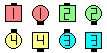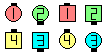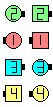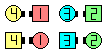Definitions of Square Dance Calls and Concepts
Central Concept [C3B]Index -->  Plus  |  A1  |  A2  |  C1  |  C2  |  C3A  |  C3B  |  C4  |  NOL  |
Definitions (Text Only) -->  Plus  |  A1  |  A2  |  C1  |  C2  |  C3A  |  C3B  |  C4  |  NOL  |
 Find call:

 \$B8@8l(Banything \$B%3!<%k\$N(B centers \$B\$N%Q!<%H\$r9T\$\$\$^\$9(B.

\$B\$b\$7;O\$a\$N(B formation \$B\$,(B 2 x 4 \$B\$G(B, anything \$B%3!<%k\$N(B centers \$B\$N%Q!<%H\$,(B 1 x 4 \$B\$H(B 2 x 2 \$B\$N\$I\$A\$i\$+\$i\$G\$b=PMh\$k\$H\$-(B (\$BNc\$(\$P(B, Counter \$B\$d(B Detour) \$B\$O(B, 2 x 2 \$B\$GF0\$-\$^\$9(B. \$B\$3\$N>l9g(B, \$B%3!<%i!<\$O%@%s%5!<\$K\$I\$A\$i\$N(B setup \$B\$GF0\$/\$+(B (\$BNc\$(\$P(B, Each Wave do a Central Counter) \$B\$r;X<(\$9\$Y\$-\$G\$9(B.

Central Spin The Windmill:Central Spin The Windmill\$B\$NA0(B Swing-Slip\$B\$N8e(B Cast Off 3/4\$B\$N8e(B (\$B=*\$o\$j(B)

Central Ease Off:Central Ease Off\$B\$NA0(B Split Circulate & 1/4 In\$B\$N8e(B (\$B=*\$o\$j(B)

C3B \$B\$G(B Central \$B\$,;H\$(\$k%3!<%k\$N0lIt\$N%j%9%H(B:

 Choice Counter Cross Counter Cross Ramble Delight | Dilemma Detour Dodge anything Ease Off Explode The Diamond Fan Chain Thru Hocus Pocus Horseshoe Turn Interlocked Counter Interlocked Little Interlocked Little More Interlocked Rally Interlocked Ramble Little Little More Load The Boat Ramble Rally Regroup Relocate Scramble Spin The Windmill Stampede Team Up Trip The Set TurnStyle

\$BCme5-\$N%3!<%k%j%9%H\$K\$O(B, \$B%3!<%k\$N4VCf(B centers \$B\$,(B centers \$B\$K5oB3\$1\$k\$b\$N\$@\$1\$r:\\$;\$F\$\$\$^\$9(B. \$BNc\$(\$P(B, Sets In Motion \$B\$d(B Strut Right | Left \$B\$G\$O(B, centers \$B\$O(B center \$B\$N(B 4 \$B?M\$G\$O=*\$o\$j\$^\$;\$s(B. \$B\$3\$l\$i\$N%3!<%k\$G\$O(B, \$BIaDL(B outside \$B\$N\$"\$k?M\$,(B center \$B\$G=*\$o\$j\$^\$9(B. \$B\$7\$?\$,\$C\$F(B, \$B\$3\$l\$i\$N%3!<%k\$N(B Central \$B\$G\$O(B, \$B\$I\$3\$G=*\$o\$k\$+\$,\$"\$\$\$^\$\$\$G\$9(B. Phantom \$B\$N\$?\$a\$K>l=j\$r;D\$7\$^\$9\$+(B?Choreography for Central ConceptComments? Questions? Suggestions?